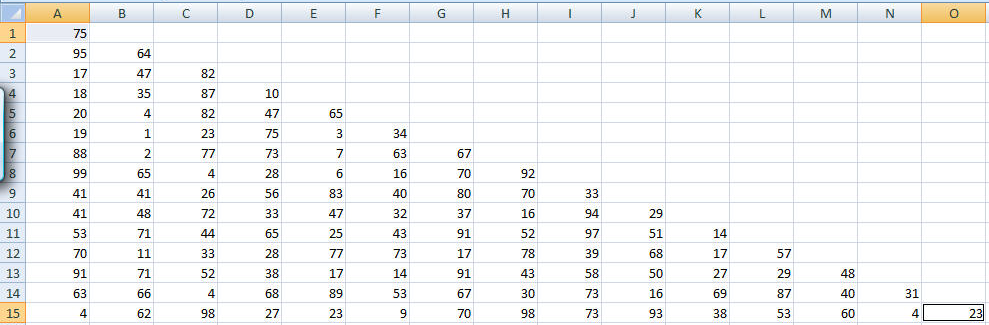﻿ Project Euler Problem 18
You are on the Home/Other Tutorials/Project Euler/Problem 18 pageShare Your

# Project Euler - Problem 18

## Problem description

By starting at the top of the triangle below and moving to adjacent numbers on the row below, the maximum total from top to bottom is 23.

3
7 5
2 4 6
8 5 9 3

That is, 3 + 7 + 4 + 9 = 23.

Find the maximum total from top to bottom of the triangle below:

75
95 64
17 47 82
18 35 87 10
20 04 82 47 65
19 01 23 75 03 34
88 02 77 73 07 63 67
99 65 04 28 06 16 70 92
41 41 26 56 83 40 80 70 33
41 48 72 33 47 32 37 16 94 29
53 71 44 65 25 43 91 52 97 51 14
70 11 33 28 77 73 17 78 39 68 17 57
91 71 52 38 17 14 91 43 58 50 27 29 48
63 66 04 68 89 53 67 30 73 16 69 87 40 31
04 62 98 27 23 09 70 98 73 93 38 53 60 04 23

NOTE: As there are only 16384 routes, it is possible to solve this problem by trying every route. However, Problem 67, is the same challenge with a triangle containing one-hundred rows; it cannot be solved by brute force, and requires a clever method! ;o)

## Solution

These kind of problems are best solved by working "backwards."  Look at row 14.  Suppose that somehow we include the 63 in our summation.  Then, which value will be pick in row 15?  Since our choices are 04 or 62, we will pick the 62.  Similarly, suppose we had reason to include the 66 in our sum.  Then, which value will be pick in row 15?  Given our choices of 62 or 98, we will pick 98.  So, we can replace the 63 in row 14 with 125 and the 66 in row 14 with 164.  We do the same with all the elements in row 14.  Now, we no longer need row 15 in our calculation.  Next, we look at the numbers in row 13.  Suppose we were to somehow pick the 91.  Then, given the new values in row 14 of 125 and 164, we would pick the 164 meaning the 91 in row 13 can be replaced by 255.  Continue this analysis all the way to row 1 and we will get the desired result.  We can implement this in Excel as follows.

Enter the triangle in a way that looks like a right-triangle but it will still be the same kind of tree as above.  For any given number, the two choices are in the row just below that number.  The first is just under the number and the other number is the one just to the right.Now, copy the last row into some other row that has 15 empty rows above it.  I chose row 35.  Then, in A34 enter the formula =MAX(A35:B35)+A14  Copy this up column A to A21:A33 and then copy A21:A34 to B:O.  One could clean up the cells so that there is only one leftmost cell in row 21, 2 leftmost cells in row 22, and so on down to 14 cells in row 34.  Or, one could leave the formulas alone and simply look at the contents of A21 since that is the answer!